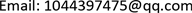1. 引言

2. 算法原理

2.1. 建立匹配窗口

1) 定位像控点：利用共线方程结合POS数据将像控点地理坐标换算以像主点为原点的像平面坐标，再将其换算为影像像素行列号，由于目标航空影像在成像时与像空间辅助坐标系存在相对旋转，因此首先将像平面坐标进行旋转变换，变换公式为：

{ x = x cos ( k ) − y sin ( k ) y = x sin ( k ) − y cos ( k ) (式1)

{ H = x + i m a g e l / 2 L = y + i m a g e c / 2 (式2)

2) 裁剪匹配窗口：以第1)步计算行列号对应像素为中心，裁剪800*800像素大小的影像作为匹配窗口，参与下一步影像匹配。

2.2. 特征点检测

M = 1 2 ( c + a − b 2 − ( a − c ) 2 )

a = ∑ ​ ( P C ( θ ) cos ( θ ) ) 2 (式3)

b = 2 ∑ ​ ( P C ( θ ) cos ( θ ) ) ∗ ( P C ( θ ) sin (θ))

c = ∑ ​ ( P C ( θ ) sin ( θ ) ) 2

2.3. 特征点匹配

1) 以特征点为中心选择100*100的图像区域作为特征区域；

2) 对特征区域进行划分，使其成为25*25的16个子区域；

3) 每个子区域统计4个方向的梯度方向直方图，16个子区域共得到64维的特征向量作为特征描述符。

dis ( k i , k j ) = 〈 k i , k j 〉 | k i | ∗ | k j | (式4)

2.4. 像控点标定

{ X = a 1 x + a 2 y + a 3 Y = b 1 x + b 2 y + b 3 (式5)

3. 实验分析3.1. 实验数据

3.2. 实验分析

1) 建立影像匹配窗口

2) 特征提取与特征匹配

3) 像控点标定

4. 结论